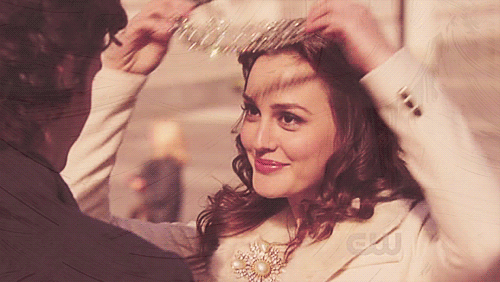WATCH: Blair Waldorf (AKA Leighton Meester) Goes From Blonde To Brunette
June 26, 2018

WATCH: Blair Waldorf (AKA Leighton Meester) Goes From Blonde To Brunette• Formula A (fill):

Redken Shades EQ 9AA + 8C + 8WG + Redken Shades EQ Processing Solution

• Formula B (lowlights):

Shades EQ 6N + 8WG + Shades EQ Processing Solution

• Formula C (base color):

L’Oréal Professionnel DIA Richesse 6.01 + 6N + 9-volume developer

• Formula D (hairline base color):

DIA Richesse 6N + 7N + 9-volume developer

• Formula E (gloss front):

Shades EQ 7NB + 8WG + 9AA + Shades EQ Processing Solution

• Formula F (gloss back):

Shades EQ 7NB + a splash of 8C + Shades EQ Processing Solution

Hey Upper East Siders BTC fam, you know there is nothing we love more than a total color transformation, and celeb colorist Justin Anderson (@justinandersoncolor) just took our fave Queen B back to her brunette roots. Yep, Leighton Meester (aka Blair Waldorf aka our favorite “Gossip Girl” cast member) just made the anything-but-simple transformation from blonde to brunette and we have all the details—like the formulas, steps and an in-process video! Check it all out below.

COLOR FORMULAS• Formula A (fill):

Redken Shades EQ 9AA + 8C + 8WG + Redken Shades EQ Processing Solution

• Formula B (lowlights):

Shades EQ 6N + 8WG + Shades EQ Processing Solution

• Formula C (base color):

L’Oréal Professionnel DIA Richesse 6.01 + 6N + 9-volume developer

• Formula D (hairline base color):

DIA Richesse 6N + 7N + 9-volume developer

• Formula E (gloss front):

Shades EQ 7NB + 8WG + 9AA + Shades EQ Processing Solution

• Formula F (gloss back):

Shades EQ 7NB + a splash of 8C + Shades EQ Processing Solution

FORMULA STEPS1. To go from blonde to brunette, the first step is to always fill the color back in. To do this, apply Formula A all over and let process. (Read more about the importance of filling color here—skip to Mistake #3!)

2. Next, rinse with Olaplex No.4 Shampoo and Olaplex No.5 Conditioner. Cleanse the hair thoroughly so that the product suds up completely.

3. Then, lowlight the hair with Formula B, leaving out the root area.

4. Apply Formula C to the base area throughout the back and side sections, then apply Formula D to the base area only along the front hairline. Let process and rinse.

5. Next, gloss the hair with Formula E in the front and Formula F in the back. Let process, rinse, blow-dry and style as desired.• Formula A (fill):

Redken Shades EQ 9AA + 8C + 8WG + Redken Shades EQ Processing Solution

• Formula B (lowlights):

Shades EQ 6N + 8WG + Shades EQ Processing Solution

• Formula C (base color):

L’Oréal Professionnel DIA Richesse 6.01 + 6N + 9-volume developer

• Formula D (hairline base color):

DIA Richesse 6N + 7N + 9-volume developer

• Formula E (gloss front):

Shades EQ 7NB + 8WG + 9AA + Shades EQ Processing Solution

• Formula F (gloss back):

Shades EQ 7NB + a splash of 8C + Shades EQ Processing Solution